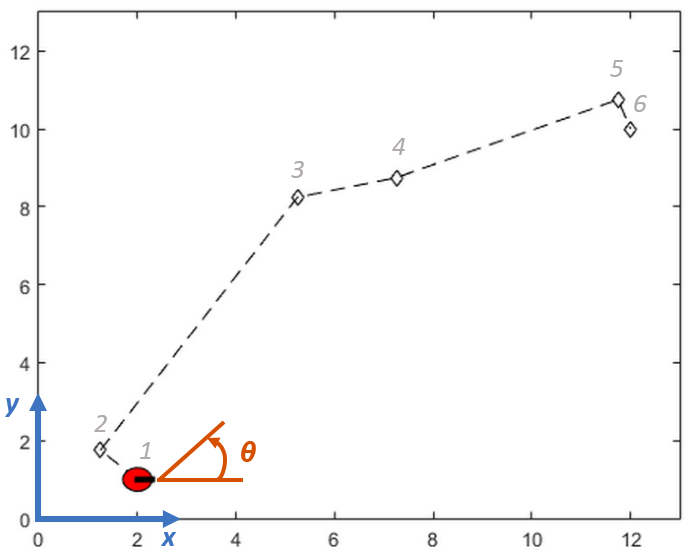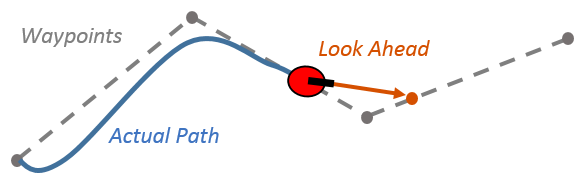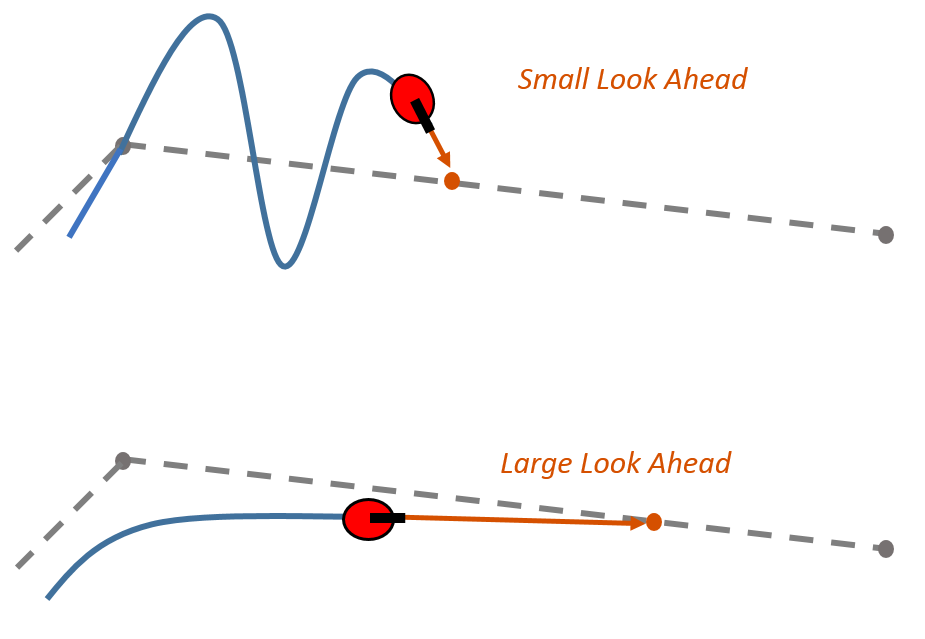## Pure Pursuit Controller

Pure pursuit is a path tracking algorithm. It computes the angular velocity command that moves the robot from its current position to reach some look-ahead point in front of the robot. The linear velocity is assumed constant, hence you can change the linear velocity of the robot at any point. The algorithm then moves the look-ahead point on the path based on the current position of the robot until the last point of the path. You can think of this as the robot constantly chasing a point in front of it. The property LookAheadDistance decides how far the look-ahead point is placed.

The `controllerPurePursuit` object is not a traditional controller, but acts as a tracking algorithm for path following purposes. Your controller is unique to a specified a list of waypoints. The desired linear and maximum angular velocities can be specified. These properties are determined based on the vehicle specifications. Given the pose (position and orientation) of the vehicle as an input, the object can be used to calculate the linear and angular velocities commands for the robot. How the robot uses these commands is dependent on the system you are using, so consider how robots can execute a motion given these commands. The final important property is the `LookAheadDistance`, which tells the robot how far along on the path to track towards. This property is explained in more detail in a section below.

### Reference Coordinate System

It is important to understand the reference coordinate frame used by the pure pursuit algorithm for its inputs and outputs. The figure below shows the reference coordinate system. The input waypoints are `[x y]` coordinates, which are used to compute the robot velocity commands. The robot’s pose is input as a pose and orientation (theta) list of points as `[x y theta]`. The positive x and y directions are in the right and up directions respectively (blue in figure). The theta value is the angular orientation of the robot measured counterclockwise in radians from the x-axis (robot currently at `0` radians).The `LookAheadDistance` property is the main tuning property for the controller. The look ahead distance is how far along the path the robot should look from the current location to compute the angular velocity commands. The figure below shows the robot and the look-ahead point. As displayed in this image, note that the actual path does not match the direct line between waypoints.The effect of changing this parameter can change how your robot tracks the path and there are two major goals: regaining the path and maintaining the path. In order to quickly regain the path between waypoints, a small `LookAheadDistance` will cause your robot to move quickly towards the path. However, as can be seen in the figure below, the robot overshoots the path and oscillates along the desired path. In order to reduce the oscillations along the path, a larger look ahead distance can be chosen, however, it might result in larger curvatures near the corners.The `LookAheadDistance` property should be tuned for your application and robot system. Different linear and angular velocities will affect this response as well and should be considered for the path following controller.

### Limitations

• As shown above, the controller cannot exactly follow direct paths between waypoints. Parameters must be tuned to optimize the performance and to converge to the path over time.

• This pure pursuit algorithm does not stabilize the robot at a point. In your application, a distance threshold for a goal location should be applied to stop the robot near the desired goal.

 Coulter, R. Implementation of the Pure Pursuit Path Tracking Algorithm. Carnegie Mellon University, Pittsburgh, Pennsylvania, Jan 1990.

﻿